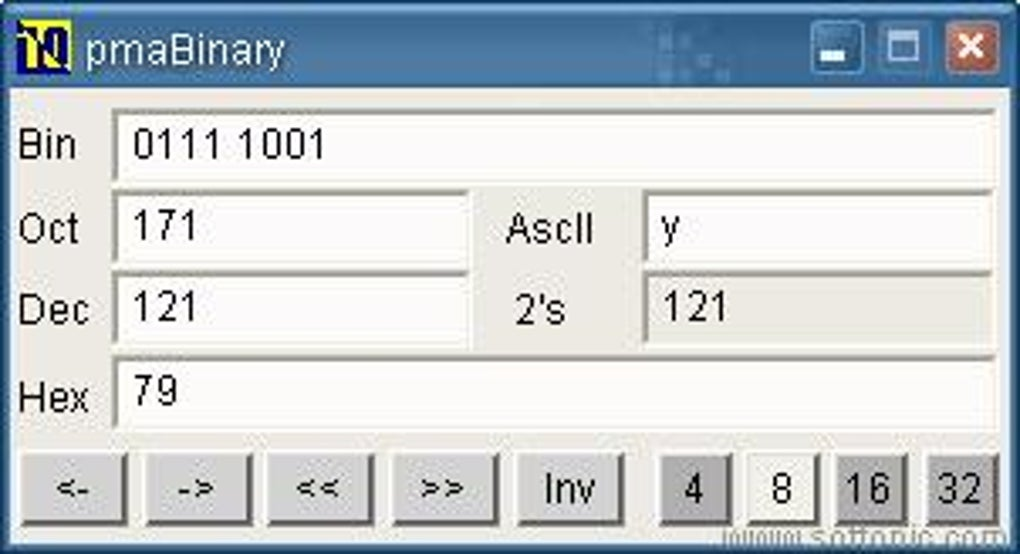### Base Conversions AssignmentProfessional software to convert between different number systems with a Graphical User Interface.
Problem: Create a program that will, given specific inputs such as the start value, starting system, and ending system, converts between decimal and your choice of binary, octal and hex (or all three). How many inputs you ask for will be determined by whether your program converts to multiple systems or not.

If you choose to do all three, you may want to separate each planned conversion into their own static methods. You may want to create a system that is based around one "intermediate system" through which all of your conversions go (similar to how we do it by hand). For example, if you have conversions for each type of system, you would get 4 system inputs (binary, octal, decimal, hex) * 4 system outputs (same) = 16 total methods.

But this kind of system is cumbersome to write and keep track of without more rigorous planning. An easier way to organize this would be to base the system around "binary", or another system of your choice, and consider that the "default case." In this scheme, if you want to convert another number, you would first convert it to binary (only three methods required b/c binary is already in binary) and then from binary to your new system (only three methods required again). This would mean that you would only write a total of six methods as opposed to sixteen different methods that, in all likelihood, would have a lot of overlap.

Many times the way you think about and organize a program will impact the complexity of the eventual implementation.

Here are some test cases for your program:

104 (Decimal) <-> 104 (Decimal)

111 (Binary) <-> 7 (Decimal)

104 (Octal) <-> 68 (Decimal)

FEC (Hex) <-> 4076 (Decimal)

This notation defines <-> to mean reversible, denoting that either the left or right sides could serve as inputs/outputs for the program.

Cases sourced from http://www.cs.trincoll.edu/~ram/cpsc110/inclass/conversions.html